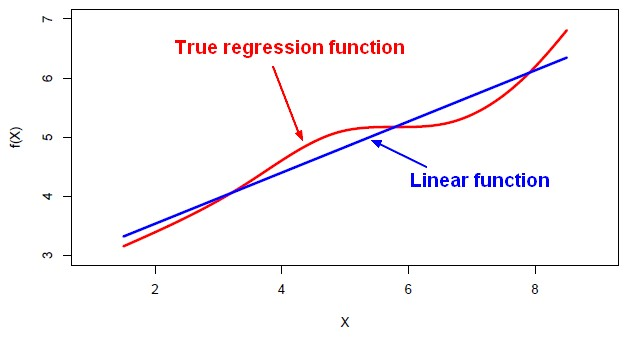# Machine Learning - Multi-response linear regression (Linear Decision trees)

The multi-response linear regression method is a non-linear methods that build trees of linear models.

## Representation

Model tree:

• Each leaf has a linear regression model

How it will choose the node?

• The Multi-response linear regression method will choose the class of an instance according to whichever of the three regression formulae produces the largest output. Ie the correlation coefficient is much smaller than for the others.

## Implementation

### Weka

Tree > m5p

Recommended PagesColorSpace (Model)

A color space (or model) is a coordinate space where a set of coordinate define an unique color.Machine Learning - Linear (Regression|Model)

Linear regression is a regression method (ie mathematical technique for predicting numeric outcome) based on the resolution of linear equation. This is a classical statistical method dating back more...